工业与基础设施

制造业• 计算器
• 摄像机
• 时钟
• 一氧化碳探测器
• 电动订书机
• 洗手液
• 办公室设备
• 投影仪遥控器
• 烟雾探测器
• 磁带录音机
• 恒温控制器
• DVD/电视遥控器
• 双向无线电
• 无线耳机
• 无线键盘
• 无线麦克风

• 警报器/传感器
• 时钟
• 气体探测器
• 测试设备
• 双向无线电

• 自动给皂器
• 自动毛巾分配器
• 汽车读码器/扫描仪
• 钳形仪表
• 电筒
• 地磅
• 便携式计时器
• 烟雾探测器
• 转速表
• 测试设备
• 胎压计
• 双向无线电

• 自动给皂器
• 自动毛巾分配器
• 时钟
• 地磅
• 食品温度计
• 手动搅拌器
• 洗手液
• 红外测温仪
• 安保摄像机
• 定时器

• 寻呼机
• 双向无线电
• 无线耳机
• 无线键盘
• 无线麦克风

• 大气监测器
• 电池和电路测试仪
• 电缆测试仪
• 摄像机
• 钳形仪表
• 时钟
• 一氧化碳探测器
• 数字温度计
• 门钥匙垫
• 门锁
• 应急灯笼
• 电筒
• 洗手液
• 安全帽灯
• 照明放大镜
• 红外测温仪
• 标签机
• 卷尺
• 金属探测器
• 寻呼机
• 折光仪
• 安保摄像机
• 探测器
• 测试设备
• 双向无线电
• 灌溉定时器

• UPC 读码器

• 时钟
• 标签机
• 作业通信/无线电
• 磅秤
• 扫描仪

• 空气质量测试仪
• 过敏原检测试剂盒
• ATP 监测器
• 自动纸巾分配器
• 天平
• 饮料测试器
• 钳形仪表
• 时钟
• 计数器
• 数字万用表
• 数字温度计
• 电路测试仪
• 紧急出口警报器
• 环保核查测试仪
• 电筒
• HAACP 监测器
• 洗手液
• 湿度计
• 湿度温度计
• 红外测温仪
• 无钥匙进入控制锁
• 金属探测器
• 模具测试仪
• pH 测试仪
• 标签机
• 打印和记录温湿度计
• 干湿表
• 制冷剂监测器
• RH 温度
• 磅秤
• 烟雾探测器
• 氯化钠计
• 转速表
• 温度/湿度记录器
• 热敏电阻
• 热电偶温度计
• 毒素探测器
• 电压探测器
• 水检测试剂盒

• 时钟
• 一氧化碳探测器
• 烟雾探测器

• 空气质量测试仪
• 数字温度计
• 计量器
• 湿度计
• 测试设备
• 定时器

• 作业通信/无线电
• 动作传感器
• UPC 读码器

• 计算器
• 摄像机
• 时钟
• 一氧化碳探测器
• 电动订书机
• 电筒
• 洗手液
• 标签机
• 金属探测器
• 办公室设备
• 削笔器
• 安全键盘
• 烟雾探测器
• 恒温控制器
• 无线耳机
• 无线键盘
• 无线麦克风

• 空气清新剂
• 自动水龙头（池内）
• 自动给皂器
• 自动马桶
• 自动毛巾分配器
• 冲洗阀
• 洗手液

• 时钟
• 标签机
• 磅秤
• 扫描仪
• 双向无线电

• 建筑计算器
• 测距仪
• 电筒
• 万用表
• 测试设备

• 计算器
• 时钟
• 电筒
• 激光指示器
• 投影仪遥控器
• 烟雾探测器
• 磁带录音机
• 无线键盘
• 无线麦克风

建筑通信（电视、电缆、电信）

• 音频分析仪
• 音频信号发生器
• 电缆分析仪
• 电缆测试仪
• 摄像机
• CATV 电缆测试仪
• 电导率测试仪
• 数字听度计
• 电筒
• GPS 设备
• 谐波分析器
• 作业通信/无线电
• 标签机
• LAN 电缆测试仪
• LED 测试仪
• 线缆测试仪
• 喇叭筒
• 微扫描仪
• 模块化电缆测试仪
• 非接触式电压探测器
• Pocket Toner CATV 电缆测试仪
• Pocket Toners
• 便携式发射器
• 电阻测试仪
• Resi-toner
• 扬声器阻抗计
• 探测器
• 电话线路测试仪
• 无接触电压指示器
• 双向无线电
• 地下电缆定位器

建筑

• Bulls Eye 激光器
• 建筑计算器
• 深度计
• 数字分度器
• 电工组合包
• 电子卡尺
• 电子水平仪
• 电筒
• GPS 设备
• 安全帽灯
• 测高仪
• 红外测温仪
• 作业通信/无线电
• 标签机
• 激光测距装置
• 激光估计器
• 激光铅锤
• 喇叭筒
• 金属探测器
• 金属定位器
• 折光仪
• 自动调平激光铅垂线
• 探测器
• 双向无线电
• 地下电缆定位器
• 视频摄像头示波器
• 墙壁传感器和扫描仪

• 电缆分析仪
• 电缆测试仪
• 电容器测试仪
• 电路测试仪
• 钳形仪表
• 电导率测试仪
• 电路测试仪
• 电筒
• 照明放大镜
• 作业通信/无线电
• 标签机
• LED 测试仪
• 测光计
• 线缆测试仪
• 万用表
• 多通道示波器
• NTSC 信号发生器
• 探测器
• 无接触电压指示器
• 双向无线电
• 地下电缆定位器
• 视频摄像头示波器
• 电压表
• 无线吊扇控制装置
• 无线调光器
• 无线烙铁

总承包方

• Bulls Eye 激光器
• 建筑计算器
• 深度计
• 数字分度器
• 电工组合包
• 电子卡尺
• 电子水平仪
• 电筒
• GPS 设备
• 安全帽灯
• 测高仪
• 红外测温仪
• 作业通信/无线电
• 标签机
• 激光测距装置
• 激光估计器
• 激光铅锤
• 喇叭筒
• 金属探测器
• 金属定位器
• 折光仪
• 自动调平激光铅垂线
• 探测器
• 双向无线电
• 地下电缆定位器
• 视频摄像头示波器
• 墙壁传感器和扫描仪

• 气流计
• 大气监测器
• 风速计
• 电缆测试仪
• 电容器测试仪
• 氯测试仪
• 电路测试仪
• 钳形仪表
• 一氧化碳探测器
• 电导率测试仪
• 数字温度计
• 电路测试仪
• 火焰安全控制测试仪
• 法兰面板温度计
• 电筒
• 气体探测器
• 硬度测试仪
• 湿度计
• HVACIR 分析仪
• 湿度温度计
• 红外测温仪
• 离子发生器测试仪
• 作业通信/无线电
• 标签机
• 兆欧表
• 微压计
• 氧测试仪
• pH 检测仪
• 制冷剂泄漏探测器
• 相对湿度计
• 热电偶温度计
• 恒温控制器
• 双向无线电
• 地下电缆定位器
• 视频摄像头示波器

• 电筒
• 气体探测器
• 作业通信/无线电
• 标签机
• 双向无线电
• 地下电缆定位器
• 视频摄像头示波器
• 灌溉定时器

实用工具• 路障灯
• 摄像机
• 耳罩
• 电筒
• 气体探测器
• GPS 装置
• 标签机
• 线缆测试仪
• 抄表器
• 测试设备
• 地下电缆定位器
• 视频摄像头示波器
• 风速指示器

• 气量计
• 大气监测器
• 电流表
• 电缆分析仪
• 电缆测试仪
• 芯片测量分析仪
• 氯测试仪
• 电路测试仪
• 一氧化碳探测器
• 电导率计
• 摄像机
• 数字温度计
• 门锁
• 耳罩
• 电子平衡器
• 电子卡尺
• 电子磅秤
• 静电定位器计
• 电筒
• 气体分析仪
• 气体探测器
• 气体流量计
• 安全帽灯
• 离子发生器测试仪
• 标签机
• 甲烷检测仪
• 万用表
• 氧测试仪
• 便携式警告灯
• 辐射报警监测器
• 烟雾探测器
• 静电测量表
• 恒温控制器
• 双向无线电
• 地下电缆定位器
• 电压表
• 电压探测器

变电所

• 空气清新剂
• 自动水龙头（池内）
• 自动给皂器
• 自动马桶
• 自动毛巾分配器
• 电缆测试仪
• 时钟
• 接地检测器
• 电筒
• 冲洗阀
• 气体探测器
• GPS 装置
• 手持式示波器
• 红外测温仪
• 电能质量测试仪
• 过程工具
• 烟雾探测器
• 测试设备
• 热像仪
• 双向无线电
• 地下电缆定位器
*以上解决方案仅供参考实际效益可能因设备制造、型号和使用频率而有所不同。

冲洗阀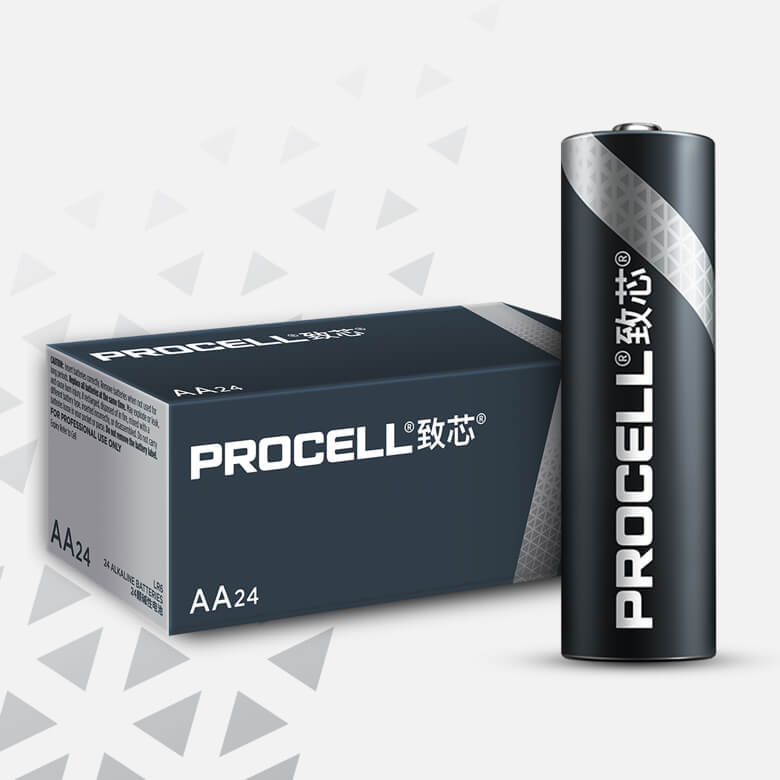Procell致芯碱性电池, AA, 1.5V

PC1500
Procell致芯碱性电池AA 工业电池为通用电池，非常适合于为中低电流专业设备提供电力，如烟雾/一氧化碳探测器、安全键盘、遥控器、无线鼠标、键盘、运动传感器和冲水阀。 另外还提供 AAA、C、D 和 9V 规格。

24

纸巾分配器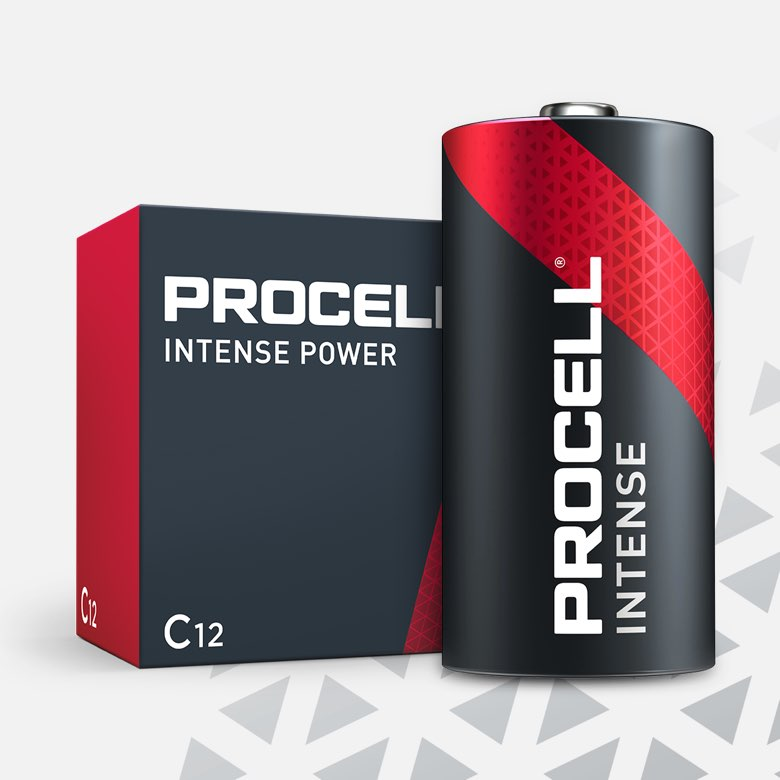Procell致芯宏耀碱性电池, C, 1.5V

PX1400
Procell C 工业用碱性强力电池专为需要高功率或峰值功率的高耗能专业设备（如皂液器）设计，与之前的 Procell 碱性 C 电池相比，使用寿命更长。还有 AA 和 D 两种规格可供选择。

12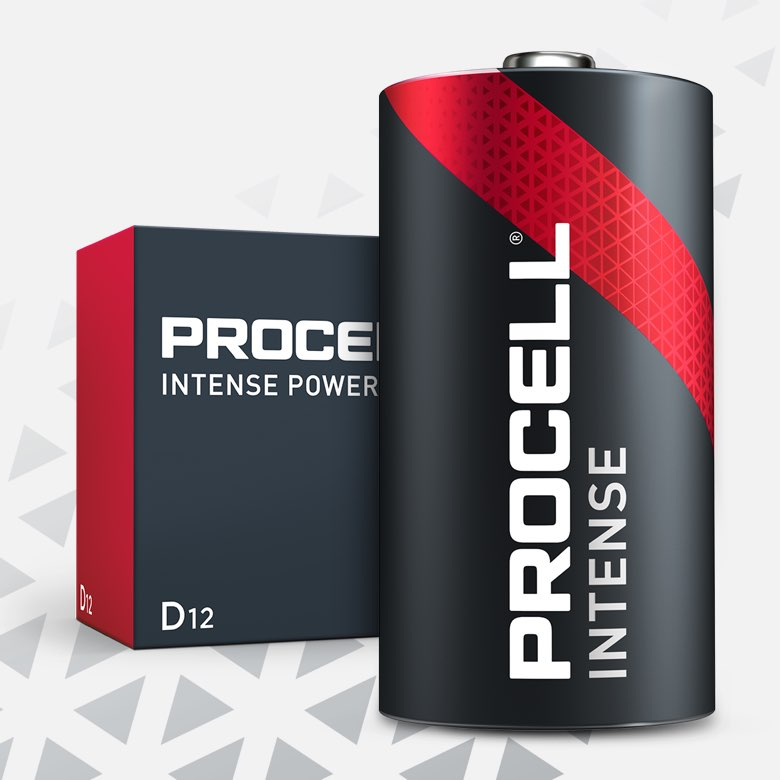Procell致芯宏耀碱性电池, D, 1.5V

PX1300
Procell D 工业用碱性强力电池专为需要高功率或峰值功率的高耗能专业设备（如纸巾分配器）设计，与之前的 Procell 碱性 D 电池相比，使用寿命更长。还有 AA 和 C 两种规格可供选择

12

肥皂分配器Procell致芯宏耀碱性电池, C, 1.5V

PX1400
Procell C 工业用碱性强力电池专为需要高功率或峰值功率的高耗能专业设备（如皂液器）设计，与之前的 Procell 碱性 C 电池相比，使用寿命更长。还有 AA 和 D 两种规格可供选择。

12

自动水龙头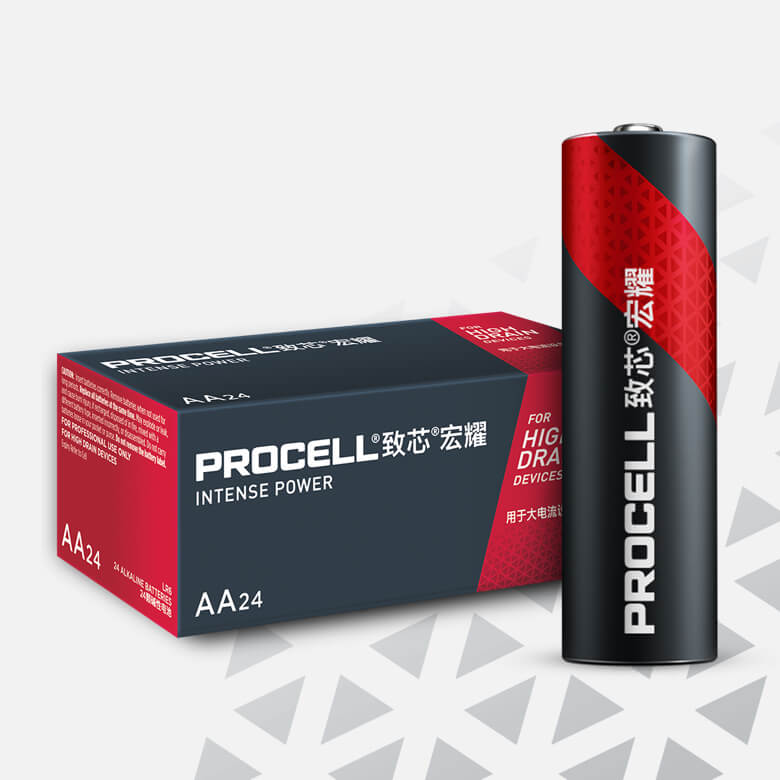Procell致芯宏耀碱性电池, AA, 1.5V

PX1500
Procell致芯宏耀碱性电池AA 工业电池专门设计用于为需要大量电力或电力峰值的大电流专业设备提供持久电力，如电子门锁、皂液器或安保摄像头。 另外还提供 C 和 D 规格。

24Procell致芯宏耀碱性电池, C, 1.5V

PX1400
Procell C 工业用碱性强力电池专为需要高功率或峰值功率的高耗能专业设备（如皂液器）设计，与之前的 Procell 碱性 C 电池相比，使用寿命更长。还有 AA 和 D 两种规格可供选择。

12Procell致芯宏耀碱性电池, D, 1.5V

PX1300
Procell D 工业用碱性强力电池专为需要高功率或峰值功率的高耗能专业设备（如纸巾分配器）设计，与之前的 Procell 碱性 D 电池相比，使用寿命更长。还有 AA 和 C 两种规格可供选择

12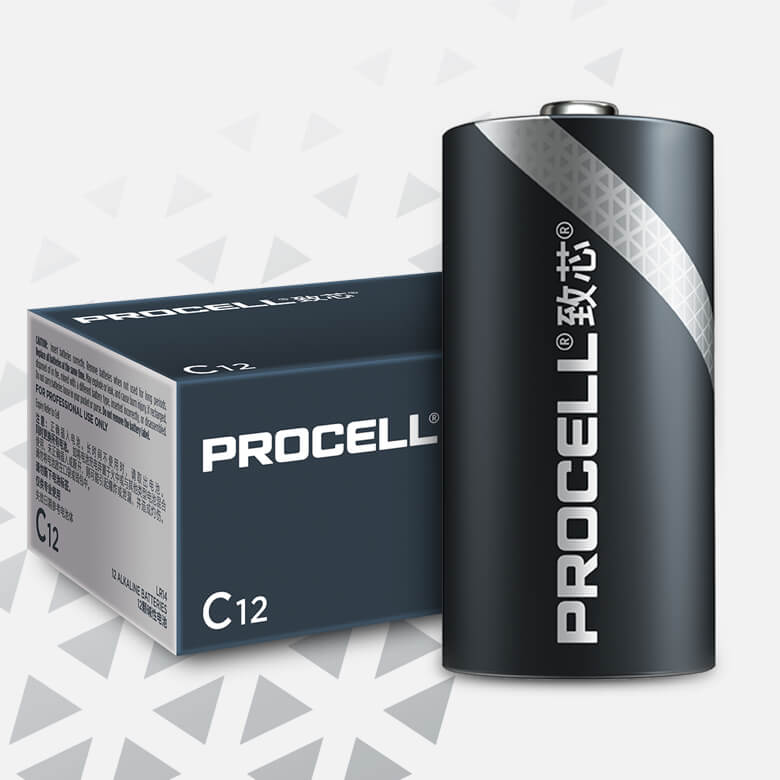Procell致芯碱性电池, C, 1.5V

PC1400
Procell致芯碱性电池C 工业电池为通用电池，非常适合于为中低电流专业设备提供电力，如安全键盘和自动水龙头。 另外还提供 AA、AAA、D 和 9V 规格。

12Procell致芯碱性电池, D, 1.5V

PC1300
Procell致芯碱性电池D 工业电池为通用电池，非常适合于为中低电流专业设备提供电力，如手电筒和传感器。 另外还提供 AA、AAA、C 和 9V 规格。

12

电动百叶窗Procell致芯宏耀碱性电池, AA, 1.5V

PX1500
Procell致芯宏耀碱性电池AA 工业电池专门设计用于为需要大量电力或电力峰值的大电流专业设备提供持久电力，如电子门锁、皂液器或安保摄像头。 另外还提供 C 和 D 规格。

24